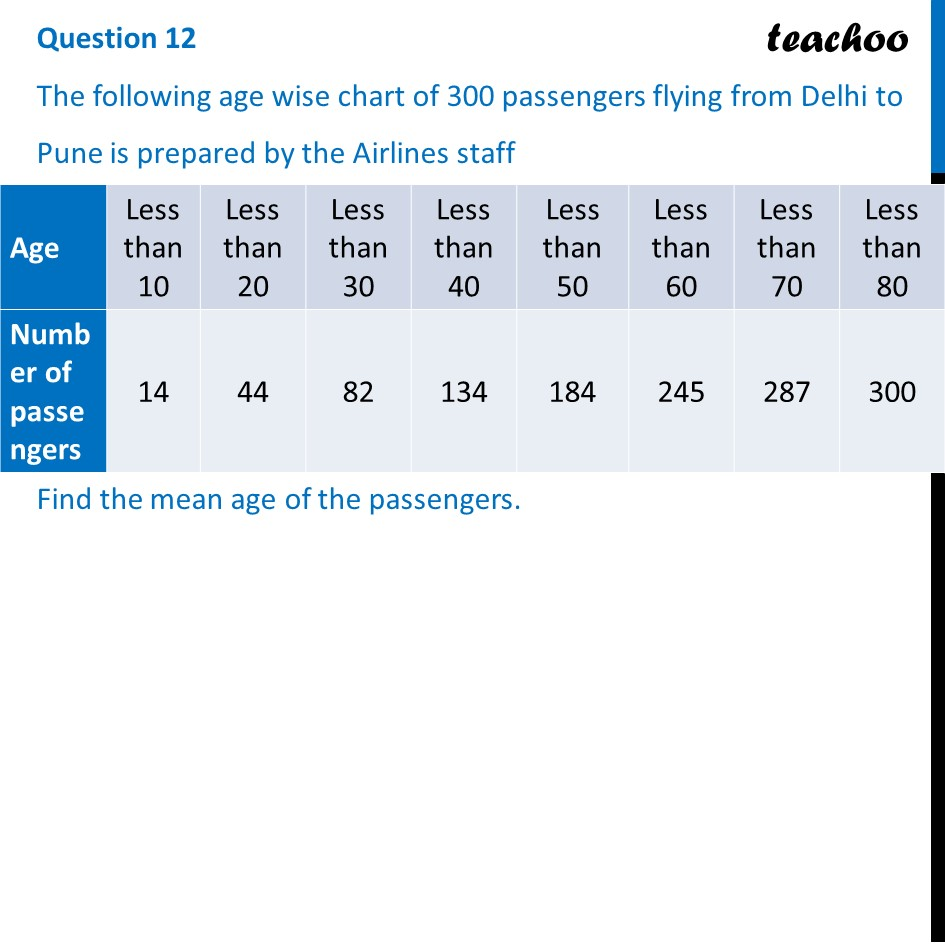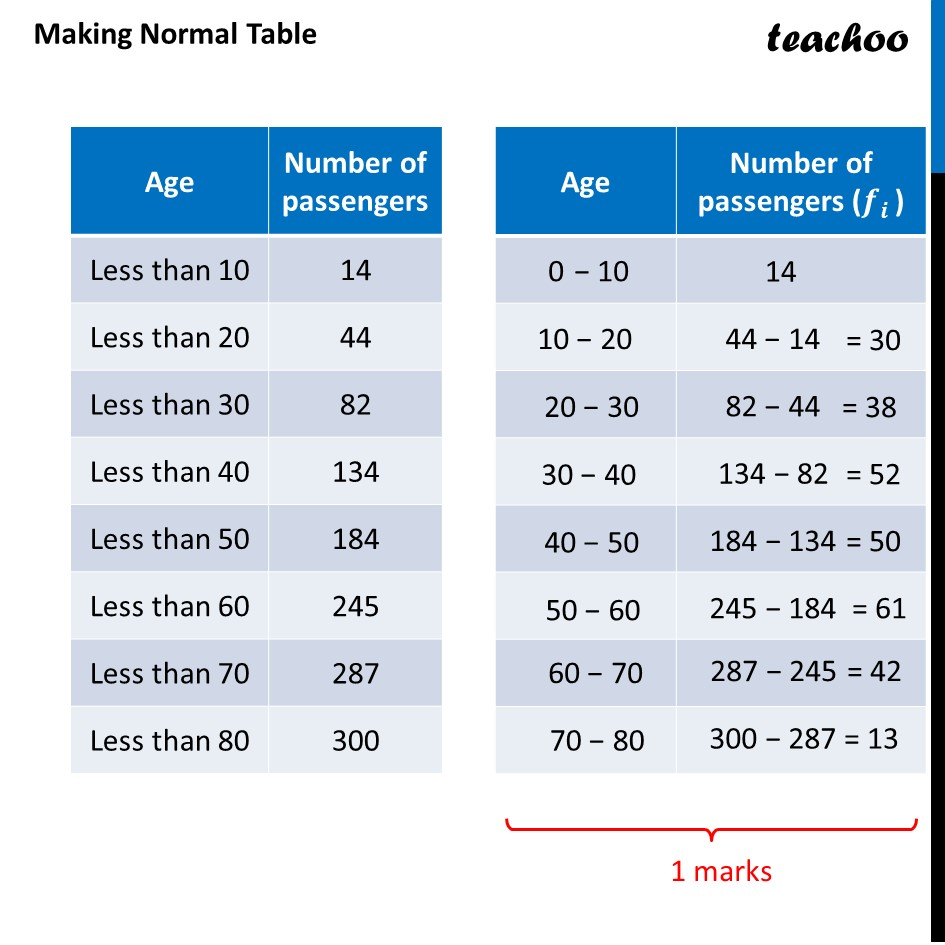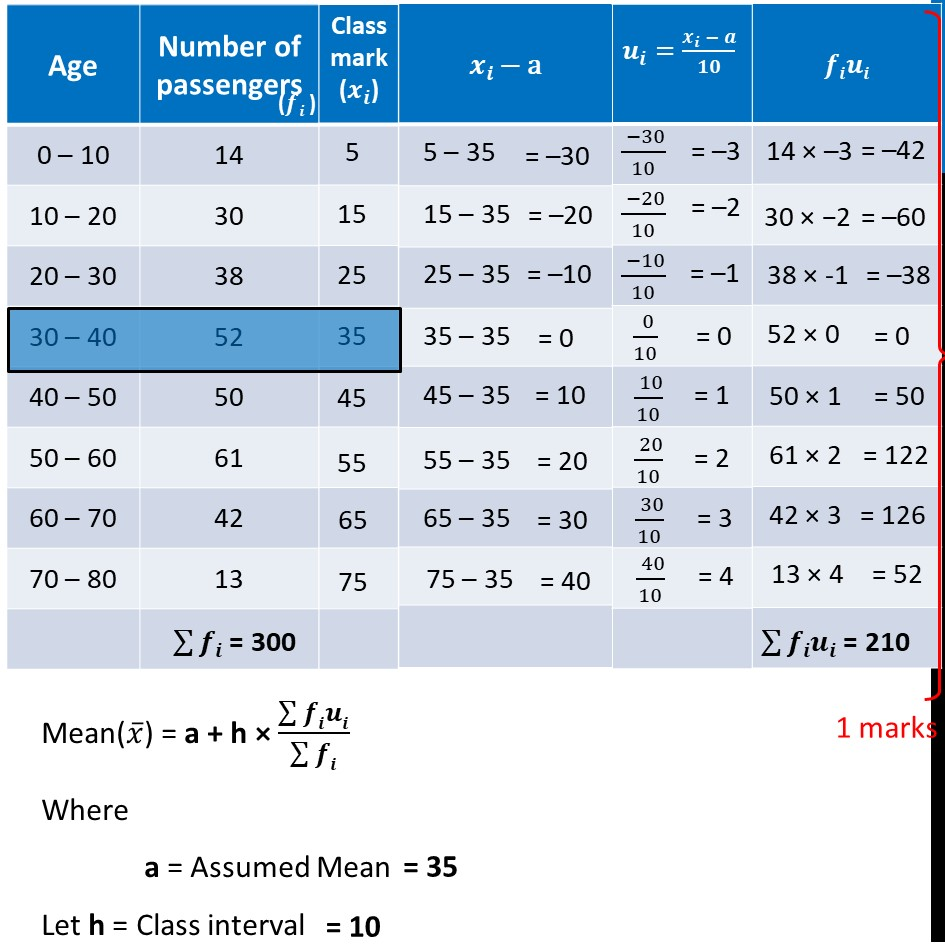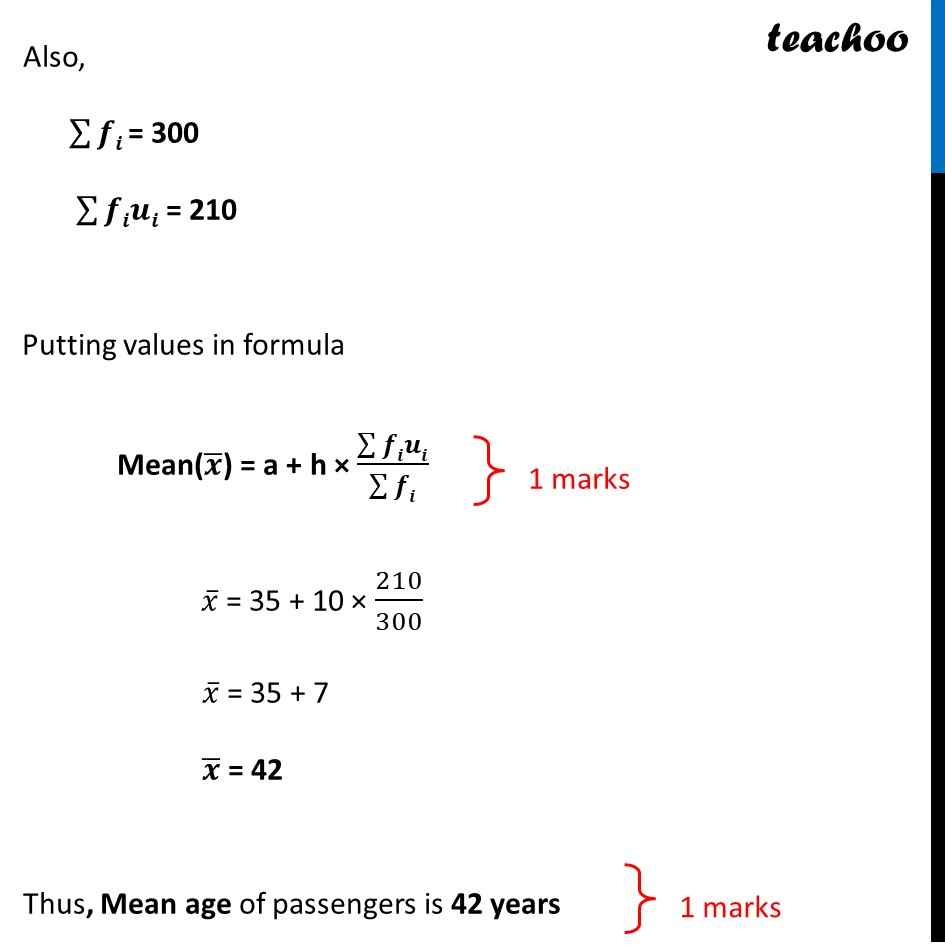CBSE Class 10 Sample Paper for 2022 Boards - Maths Basic [Term 2]

Class 10
Solutions of Sample Papers for Class 10 Boards

## Find the mean age of the passengers.

This question is similar to Example 7 Chapter 14 Class 10 - StatisticsGet live Maths 1-on-1 Classs - Class 6 to 12

### Transcript

Question 12 The following age wise chart of 300 passengeMaking Normal Table rs flying from Delhi to Pune is prepared by the Airlines staff Find the mean age of the passengers. Mean(𝑥 ̅) = a + h × (∑▒𝒇𝒊𝒖𝒊)/(∑▒𝒇𝒊) Where a = Assumed Mean Let h = Class interval Also, ∑▒𝒇𝒊 = 300 ∑▒𝒇𝒊𝒖𝒊 = 210 Putting values in formula Mean(𝑥 ̅) = a + h × (∑▒𝒇𝒊𝒖𝒊)/(∑▒𝒇𝒊) Where a = Assumed Mean Let h = Class interval Also, ∑▒𝒇𝒊 = 300 ∑▒𝒇𝒊𝒖𝒊 = 210 Putting values in formula Mean(𝒙 ̅) = a + h × (∑▒𝒇𝒊𝒖𝒊)/(∑▒𝒇𝒊) 𝑥 ̅ = 35 + 10 × 210/300 𝑥 ̅ = 35 + 7 𝒙 ̅ = 42 Thus, Mean age of passengers is 42 years